SCHOLASTIC APTITUDE TEST 1996

MATHEMATICS

 Time: Two Hours Max. Marks: 60 (8.30 AM - 10.30 AM)

NOTE:-

• Answers must be written in English or the medium of instruction of the candidate in High school.
• There are nine questions in this paper. Answer to each question should begin on a fresh page, and rough work must be enclosed to answer book.
• Attempt all questions.
• There is no negative marking.
• Answer all parts of a question at one place.
• Use of calculators, slide rule, graph paper and logarithmic, trigonometric and statistical tables is not permitted

PART-A

I. This section contains (7) questions. Each question has one or more than one correct answer(s). Indicate the answer(s) by A,B,C,D with brief reasoning. Order of the questions must be maintained.                                       (7 x 2 =14 MARKS)

1. Let n be a product of four consecutive positive integers then
 A) n+1 is always a perfect square B) n is never a perfect square C) n is always divisible by 24 D) n+12 is always a perfect square
1. log(x+3)+log(x-1)=log(x2-2x-3) is satisfied for
 A) All real values of x B) No real value of x C) All real values of x except x=0 D) No real value of x except x=0 E) All real values of x except x=1
1. |sinx|tanx > |sinx|cotx            then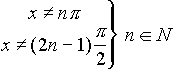A)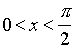B)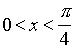C)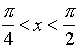D) None of these
 Which of the two functions are equivalent A)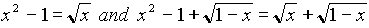B)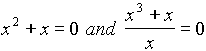C)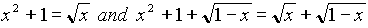D)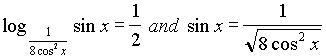In a traingle ABC, the sides are in the ratio 1:1: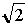it follows that [ ] A) Angles of the traingle are in ratio 1:1:2 B) Angles of the traingle are in the ratio 1:2:3 C) The traingle is right angle traingle D) The circum circle of the D ABC has its centre on one of the sides
1. vi. There are exactly 4 prime numbers between n and 2n. Then possible value of n is
 A) n=4 B) n=10 C) n=12 D) n=50
2. The relation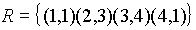on the X={1,2,3,4}
 A) not a function from X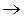X B) an one to one function from XX C) an onto function of XX D) R is a transitive relation on X

II. This section contains (6) in-complete statements. complete the statements by filling the blanks. Write your answers in your answer book in the order in which the statements are given below. (6 x 2=12 MARKS)

i. If for some angle q and for some real number x it is given that sinq = x2-2x+2 then x should be equal to _______________ and q should be equal to ________________ degrees

ii. If a, b, c, d are four distinct integers between 1 to 10 such that for a traingle whose sides are in the ratios a:b:c, the altitudes are d:c:b. Then greatest of these four integers is _____________ and the smallest is __________________

iii. In a cylinder, AB is a diameter of the base circle and C is the centre of the top circle. Given that the volume of the cylinder is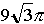cm3.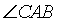= 300, the perimeter of the traingle ABC is _____________ and the curved surface area of the cone with vertex C (having the same base) is ______________

iv. If the sum of the intercepts of the line with coordinate is 5 and the area of the traingle formed by the line and coordinate axes is 3 then intercepts are ______________________

v. In the figure given below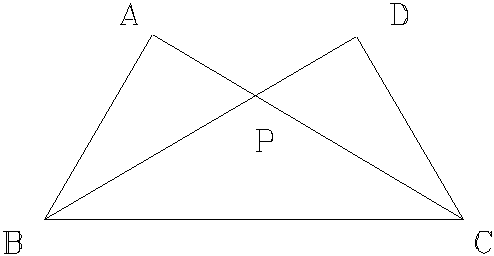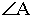=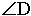= 900 AP = 7.5 cm, AB = 10 cm, BC = 26 cm, Then CD is ________

vi.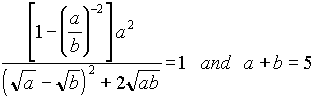Then a, b are __________________

III. This section contains (8) statements in GROUP-A and (4) questions in GROUP-B. Answer questions in GROUP-B on the basis of the statements made in GROUP-A. (4 x 1 = 4 MARKS)

GROUP-A

A,B and C are three distinct points in a plane .

i . There is no point D equidistant from A,B,C

ii. B lies on the perpendicular bisector of AC

iii. Among the three lengths AB, BC, AC the largest is the sum of the other two

iv. There is a point D such that ABCD is a rhombus

v. There is a point D such that ABCD is a rectangle

vi. In triangle ABC, the angular bisector B is perpendicular to the side AC

vii. AB is a tangent to the circle through B with centre C

viii. AC is a diameter of the circle passing through A, B, C.

GROUP-B

1. Which of the above statements mean that A, B, C are collinear

2. Which of the above statements mean AB = BC

3. Which of the above statements mean that AB is perpendicular to BC

4. Which of the above statements infer ABCD is cyclic quadrilateral

PART-B

• Attempt all questions

IV. The numerator of a fraction is less than its denominator by 2. When 1 is added to both the numerator and denominator we get another fraction. The sum of these two fractions is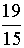. Find the first fraction .                 (5)

V. Let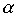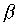be the roots of the quadratic equation x2+ax+b=0 and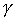,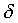be the roots of the equation

x2-ax+b-2=0. Given that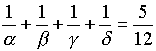and= 24. Find the value of the coefficient a.                    (5)

VI. Find the number of points with integral coordinates in the cartesian plane satisfying the inequalities

|x|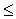100; |y|100; |x-y|100                    (5)

VII. Given an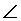ABC of magnitude less than 1800 and O is a point inside it. Draw a straight line through O which forms a traingle with minimum perimeter. (Describe the construction and give proof).                   (5)

VIII. Perpendiculars are drawn from the vertex of the obtuse angle of a rhombus to its sides. The length of each perpendicular is equal to `a’ units. The distance between their feet being equal to `b’ units. Determine the area of the rhombus. (5)

IX. Let the sequence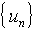be defined by

(a) u1 = 5 and the relation un+1 - un = 3 + 4(n-1) n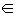N

Express un as a polynomial in n.

(b) The remainder R, when x100 is divided by x2-3x+2, is a polynomial. Find x.(3)